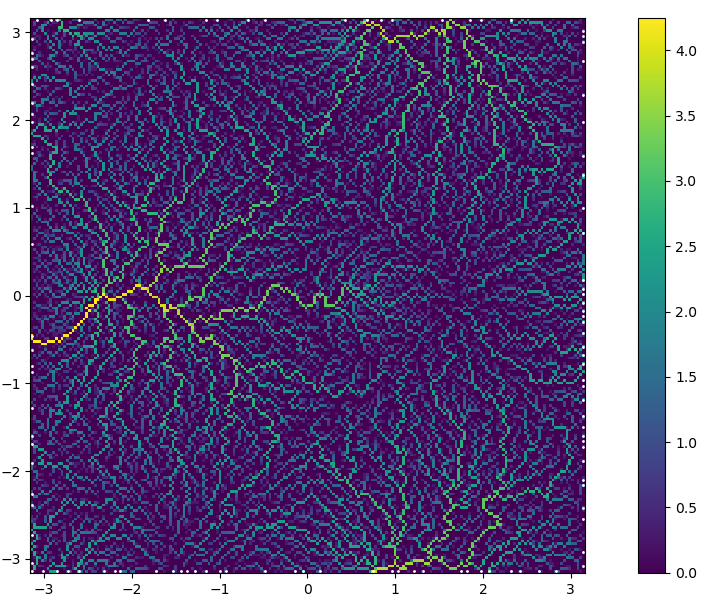## WhereTheWaterFlows.jl

Water flow on digital elevation models
Author mauro3
Popularity
8 Stars
Updated Last
1 Year Ago
Started In
October 2019

# WhereTheWaterFlows

This package implements the D8 flow routing algorithm  as well as a basin-filling algorithm, also by . It uses a O(n), recursive algorithm similar to . This allows to calculate water pathways on a digital elevation model (DEM).

This code is reasonably fast: flow routing on a DEM of Antarctica of about 2e8 points and with 150000 depressions takes about 30s on my laptop (Ryzen 4750U).Example of upslope area calculated in below example.

## Manual

```using WhereTheWaterFlows, PyPlot
const WWF = WhereTheWaterFlows

"Synthtic DEM with a few maxs and mins"
function peaks2(n=100, randfac=0.05)
coords = range(-pi, pi, length=n)
return coords, coords, sin.(coords) .* cos.(coords') .-
0.7*(sin.(coords.+1) .* cos.(coords')).^8 .+
randfac*randn(n,n)
end
x,y,dem = peaks2(200)
area, slen, dir, nout, nin, pits, c, bnds = waterflows(dem)

# log-upslope area as well as pits (sinks)
WWF.plotarea(x, y, area, pits)

# log-upslope area over contours of the dem
WWF.plotarea_dem(x, y, dem, area, pits)

# catchments
figure()
WWF.heatmap(x,y,c)

# A single catchment of some point.  Choose one with large catchment:
i, j = 50, findmax(area[50,:])
cc = catchment(dir, CartesianIndex(i,j))
WWF.heatmap(x,y,cc)
plot(x[i], y[j], "<r", ms=10)

# stream length
figure()
WWF.heatmap(x,y,slen)

demf = fill_dem(dem, pits, dir)
# "lake-depth"
figure()
WWF.heatmap(x,y,demf.-dem)```

# References

 O’Callaghan, J. and Mark, D.: The extraction of drainage networks from digital elevation data, Comput. Vision Graph., 28, 323–344, 1984. link

 Braun, J. and Willett, S.D.: A very efficient O(n), implicit and parallel method to solve the stream power equation governing fluvial incision and landscape evolution panel doi

### Used By Packages

No packages found.